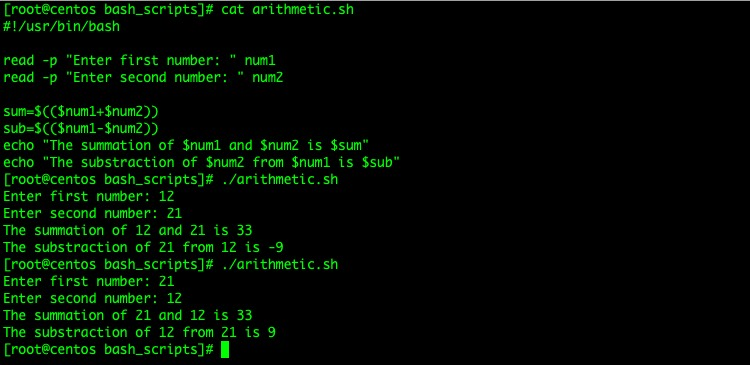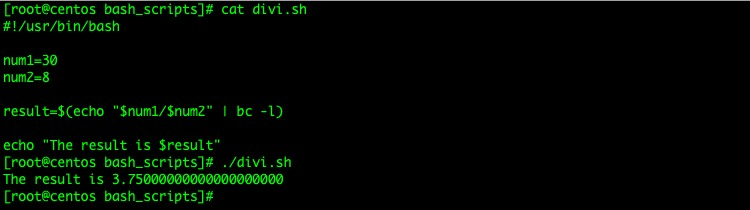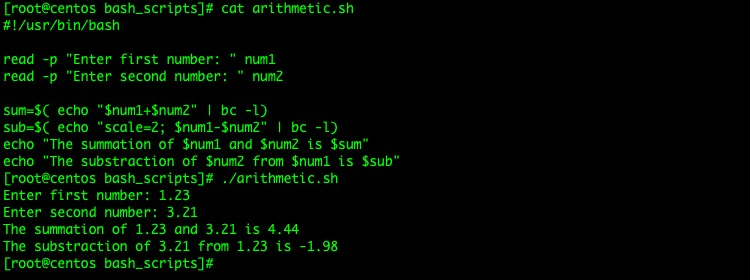Bash 脚本还可以做很多的事，比如通过变量实现简单的数学运算。

`\$((arithmetic_operation))`

`sum=\$((\$num1 + \$num2))`

Bash 支持的数学运算符

+ 加（求和）

* 乘（求积）
/ 整数除（求整数商）
% 求余
** 指数运算（求a的b次方）

PS：Bash 不支持小数，如果必须使用小数，则可以使用”bc”命令来处理它们。

```#!/bin/bash

read -p "Enter first number: " num1
read -p "Enter second number: " num2

sum=\$((\$num1+\$num2))
sub=\$((\$num1-\$num2))
echo "The summation of \$num1 and \$num2 is \$sum"
echo "The substraction of \$num2 from \$num1 is \$sub"````# echo "\$num1/\$num2" | bc -l`

```#!/usr/bin/bash

num1=30
num2=8

result=\$(echo "\$num1/\$num2" | bc -l)

echo "The result is \$result"```bc 命令的”-l”参数表示加载标准的数学库。默认情况下，”bc”命令会保留小数点后20位，我们可以向”bc”命令传递”scale”参数来指定小数点后的位数，示例如下：

`result=\$(echo "scale=3; \$num1/\$num2" | bc -l)`

Bash 脚本处理小数

```#!/usr/bin/bash

read -p "Enter first number: " num1
read -p "Enter second number: " num2

sum=\$( echo "\$num1+\$num2" | bc -l)
sub=\$( echo "scale=2; \$num1-\$num2" | bc -l)
echo "The summation of \$num1 and \$num2 is \$sum"
echo "The substraction of \$num2 from \$num1 is \$sub"```